# How to Count Dates by Day of Week in Excel

This post will guide you how to count days of week in the range of dates using a formula in Excel 2013/2016 or Excel office 365. How do I count dates by day of week in Excel.

## Count Dates by Day of Week Through Formula

Sometimes, you may be want to count days of week in a given date range or between two dates. And you need to build a complex formula to work with your data. To count dates by weekday(such as: Mondays, Tuesdays, etc), and you can use the SUMPRODUCT function in combination with the WEEKDAY function to create a formula in Excel.

For example, your date range is B1:B6, and you want to count dates by weekday, just using the following formula:

=SUMPRODUCT(–(WEEKDAY(\$B\$1:\$B\$6)=D1))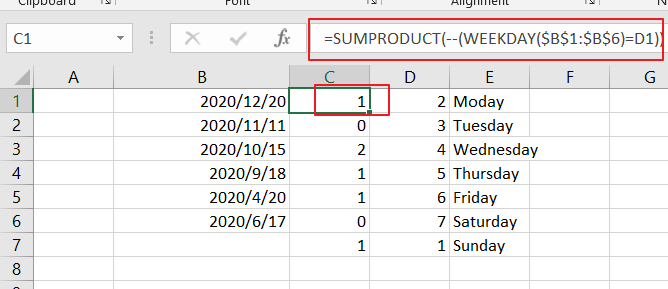LETS SEE THAT HOW THIS FORMULA WORKS:

=WEEKDAY(B1：B6)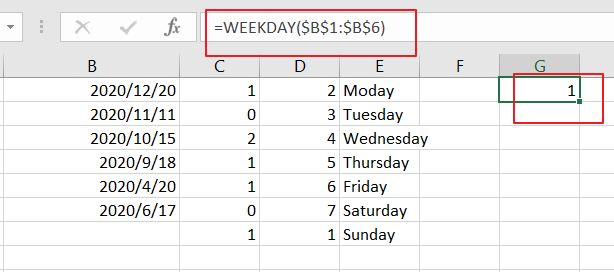The formula WEEKDAY returns a number between 1 and 7 based on a particular day of the week. Such as: Sunday is 1, Monday is 2, Thuesday is 3, Wednesday is 4, Thursday is 5, Friday is 6 and Saturday is 7.

This formula returns an array like this:

{1;4;5;6;2;4}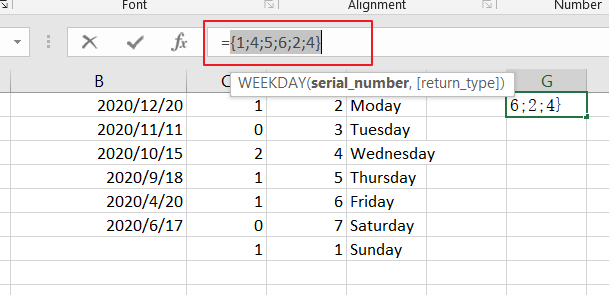=WEEKDAY(\$B\$1:\$B\$6)=1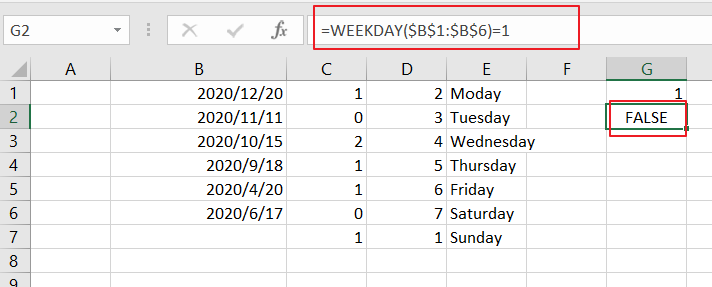Then the numbers returned by WEEKDAY formula are compared to the given day value of weekday, returns another array result like this:

{TRUE;FALSE;FALSE;FALSE;FALSE;FALSE}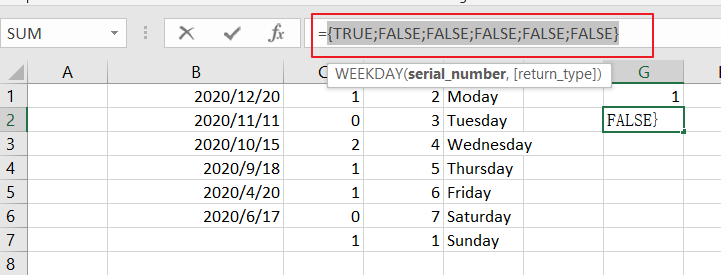=–(WEEKDAY(\$B\$1:\$B\$6)=1)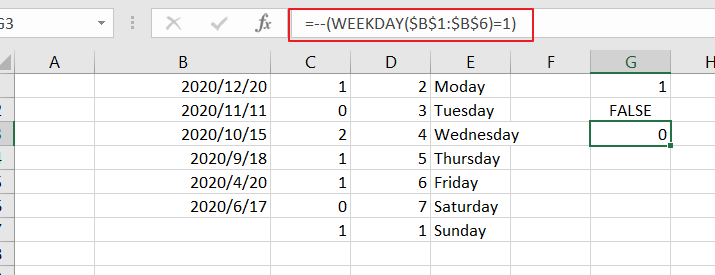You still need to convert the TRUE or FALSE values to 1 and 0 values using the double negative operator. And returns the below array result:

{1;0;0;0;0;0}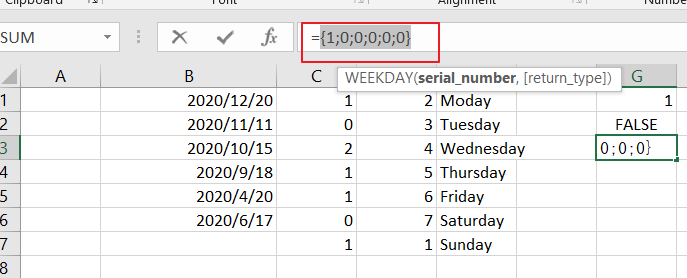The SUMPRODUCT function just sums all values of an array.

### Related Functions

• Excel SUMPRODUCT function
The Excel SUMPRODUCT function multiplies corresponding components in the given one or more arrays or ranges, and returns the sum of those products. The syntax of the SUMPRODUCT function is as below:= SUMPRODUCT (array1,[array2],…)…
• Excel WEEKDAY function
The Excel WEEKDAY function returns a integer value representing the day fo the week for a given Excel date and the value is range from 1 to 7.The syntax of the WEEKDAY function is as below:=WEEKDAY (serial_number,[return_type])…

Related Posts

How to Sum by SUMPRDUCT with One Specific Criteria Multiple Columns in Excel

Sometimes we may meet the case that to sum numbers based on one specific criteria. In this article, we will show you the method to resolve this problem by formula with the help of Excel SUMPRODUCT function. SUMPRODUCT can filter ...

How to Sum by SUMPRDUCT with Specific Criteria in Excel

Sometimes we may meet the case that to sum numbers based on two or more specific criteria. In this article, we will show you the method to resolve this problem by formulas with the help of Excel SUMPRODUCT function. SUMPRODUCT ...

How to Count Unique Numeric Values in a Range in Excel

This post will guide you how to count unique numeric values in the given range in Excel 2013/2016 or Excel office 365. How do I count the unique numeric values in a list of data with some duplicate values using ...

How to Count Unique Dates in Excel

This post will guide you how to count unique dates in a given range in Excel 2013/2016 or Excel office 365. How do I count the unique dates in a list of dates with some duplicate dates using a formula ...

How to Count Total Matches in Two Ranges in Excel

This post will guide you how to count total matches in two given ranges in Excel 2013/2016 or Excel office 365. How do I count compare two ranges and count total matches between two given ranges in Excel.And you can ...

Sidebar